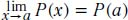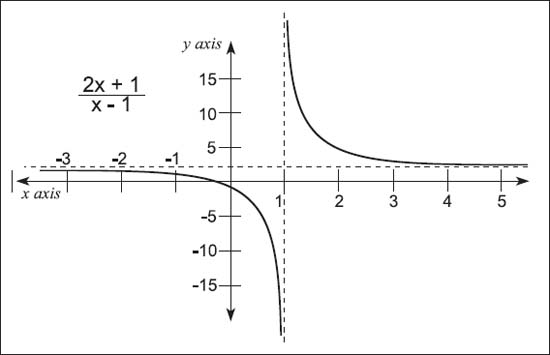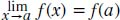# What does continuous and discontinuous mean in calculus?

When talking about polynomial functions, we know that a polynomial function P(x) satisfies the limit function:in which a represents all real numbers. This is called continuity.A graphed equation limited by horizontal asymptote y = 2.

But if f(x) is a function on an interval around a, then f(x) is continuous at a iff (see below for the definition of “iff”):if it is not, then f(x) is called discontinuous at a.

Close

This is a web preview of the "The Handy Math Answer Book" app. Many features only work on your mobile device. If you like what you see, we hope you will consider buying. Get the App